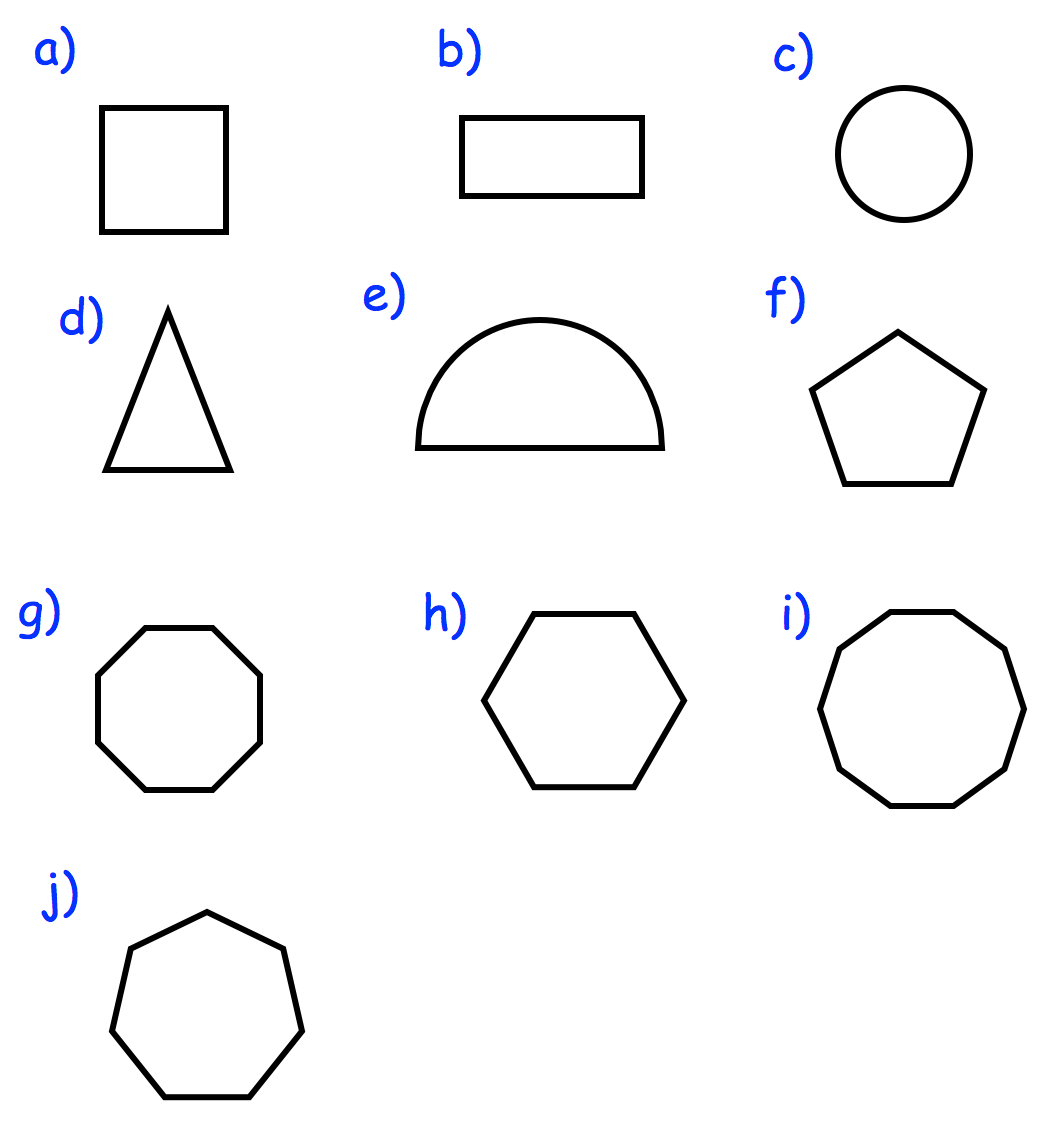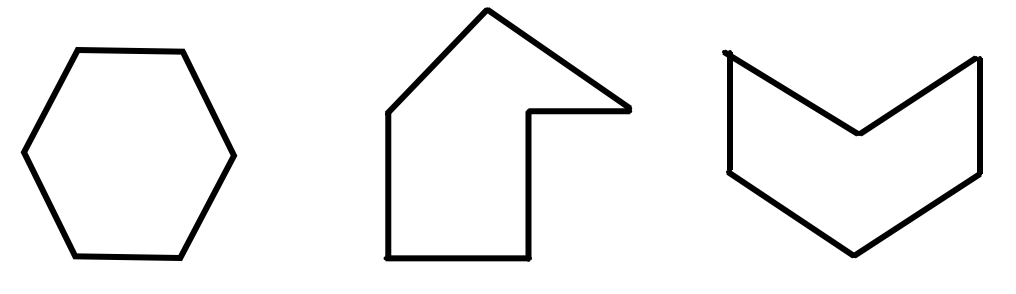Home > Uncategorized > Textbook Answers – 2D Shapes

## Textbook Answers – 2D Shapes

July 16, 2016

Worksheet – Question 1:Question 2

(a)  Rectangle

(b)  Hexagon

(c)  Triangle (right angle)

(d)  Circle

(e)  Pentagon

(f)  Square

(g)  Octagon

(h) Semi-circle

Question 3

(a)  Pentagon

(b)  Octagon

(c)  Heptagon

Apply – Question 1Question 2: (There are many options, I have listed some possible answers)

Sun – Circle

Sun rays – Triangles

Stop sign – Octagon

Hula Hoop – Circle

Bus tyres – Circles

Garage door – rectangles

Question 3

(a)  Correct

(b)  Correct

(c)  Wrong – hexagon

(d)  Wrong – parallelogram (there is no such shape as a diamond!)

Categories: Uncategorized